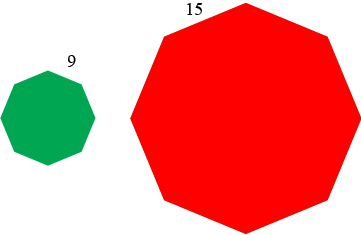SEARCH HOMEMath Central Quandaries & QueriesQuestion from tonya, a parent: What is the scale factor for the two figures regular octagon with 15 and 9 written in decimal formHi Tonya,

I imagine an image that is something like his.I am going to assume that the numbers 9 and 15 are the lengths of the sides of the respective octagons. A scale factor is between lengths so going from the smaller octagon to the larger the scale factor would be the number $k$ so that $9 \times k = 15.$ Hence

$k = \frac{15}{9} = \frac{5}{3}.$

Let me know if my assumption is incorrect.

PennyMath Central is supported by the University of Regina and the Imperial Oil Foundation.